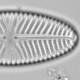## How to calculate percent from counts in R

Micropaleontologists and others often want to calculate percent from count data. From looking at archived data, I realise that what should be an easy process goes wrong far more often that it should (which is of course never). Yesterday, I found that a recently published paper had archived percent data that were impossible in several ways. Rather than discussing that paper, which has certain interesting aspects, today I am going to show how to calculate percent from count data in R.

### Case one

The counts, and only the counts, are in a species x sites (columns x rows) matrix or data.frame. Here I use the BCI count data from the vegan package.

```data(BCI, package = "vegan")
```

To calculate percent, we need to divide the counts by the count sums for each sample, and then multiply by 100.

```BCI_percent <- BCI / rowSums(BCI) * 100
```

This can also be done using the function `decostand` from the vegan package with `method = "total"`.

### Case two

The counts are in a species x sites matrix or data.frame along with other data or meta data. Here, I add the row ID from BCI to represent sample names.

```library("tidyverse")
BCI_plus <- BCI %>% rowid_to_column(var = "siteID")
```

There are a few different solutions to this. One solution is to select the columns with the count data, and process these as above. This is usually fine because we need the pure species x sites data.frame for ordinations etc

```BCI_species <- BCI_plus %>%
select(-siteID) # remove siteID column

BCI_percent <- BCI_species / rowSums(BCI_species) * 100
```

Alternatively, if we want to keep all the data in one object, we can gather the data into a thin (tidy) format, and calculate percents on that.

```BCI_percent2 <- BCI_plus %>%
gather(key = taxon, value = count, -siteID) %>% #make into thin format
group_by(siteID) %>% #do calculations by siteID
mutate(percent = count / sum(count) * 100)

head(BCI_percent2 %>% filter(count > 0))# show some data
```
```## # A tibble: 6 x 4
## # Groups: siteID 
## siteID taxon count percent
##
## 1 10 Abarema.macradenia 1 0.2070393
## 2 28 Vachellia.melanoceras 2 0.5167959
## 3 32 Vachellia.melanoceras 1 0.2178649
## 4 34 Acalypha.diversifolia 1 0.2237136
## 5 40 Acalypha.diversifolia 1 0.2044990
## 6 28 Acalypha.macrostachya 1 0.2583979
```

We can convert this thin object back to a fat format with `spread` (the opposite of `gather`). This could be appended directly onto the previous code.

```BCI_percent3 <- BCI_percent2 %>%
select(-count) %>%
spread(key = taxon, value = percent)
```

### Case three

So far, this has assumed that all the taxa are part of a single count sum as will typically be the case for diatoms, chironomids, or planktic foraminifera. With pollen, however, there can be complexities, for example, we might want to have trees, shrubs and upland herbs to be in the terrestrial pollen sum (T + S + H), and aquatic macrophytes part of the total pollen sum (T + S + H + A).

The BCI tree data obviously doesn’t have any aquatic macrophytes, but I’m going to treat all species beginning with ‘A’ as aquatic, ‘T’ as tree, ‘S’ as shrub and other taxa as herbs. First we need a dictionary, which you would normally have already.

```dictionary <- data_frame(taxon = names(BCI), group = substring(taxon, 1, 1)) %>%
mutate(group = if_else(group %in% c("A", "S", "T"), group, "H"),
sum = case_when(
group == "A" ~ "Aquatic",
group %in% c("T", "S", "H") ~ "Terrestrial"))
```

One solution is to separate the different groups into separate data.frames, calculate percent, and then bond the data.frames back together.

```aquatics <- BCI %>%
select(one_of(dictionary %>%
filter(group == "A") %>%
pull(taxon)
))

upland <- BCI %>%
select(one_of(dictionary %>%
filter(group %in% c("S", "T", "H")) %>%
pull(taxon)
))

BCI_percent4 <- bind_cols(
upland / rowSums(upland),
aquatics / rowSums(BCI)) * 100
```

And of course there is a tidyverse solution (there might even be a good tidyverse solution).

```BCI_percent5 <- BCI %>%
rowid_to_column(var = "siteID") %>% #need identifier
gather(key = taxon, value = count, -siteID) %>%
left_join(dictionary) %>%
group_by(siteID) %>%
mutate(total_count_sum = sum(count)) %>%
group_by(siteID, sum) %>%
mutate(
count_sum = sum(count),
relevant_count_sum = if_else(sum == "Aquatic", total_count_sum, count_sum),
percent = count/relevant_count_sum * 100)
```

From this object, the percent, taxon and siteID can be selected and then spread. The tidyverse solution is complicated because the count sums overlap.Ecologist with interests in quantitative methods and palaeoenvironments
This entry was posted in Data manipulation, R. Bookmark the permalink.

### 2 Responses to How to calculate percent from counts in R

1.Yuniel says:

Lovely post! I have a mix solution using a function and dplyr and combining your own solutions.
#use your code line to create a function for percentage calculation
Percentage.calc%
select(5:10) %>% #select desired numeric columns, alternatively you can do “select_if(is.numeric)”